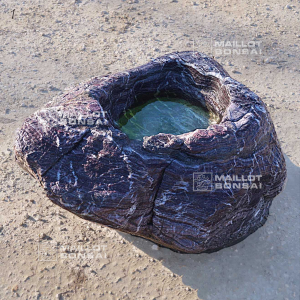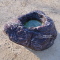##### The Japanese Bonsai specialist
Direct order Contact Help / Services Newsletter# Bassin tsukubai samba n°1

› Lanterns, basins and statues › Water basin, mizu bachi, tsukubaref. : 9040

1.125,00

voluminous/heavy item extra shipping of 65,00

This item is temporarily unavailableNotify availability

###### Description

Samba rock imported from Japan. Unique piece, natural with its veins.
This rock is used to create chaniwa, japanese gardens, tea gardens or walk gardens. This model has a central hole that retains water. It is one of the elements composing the tsukubai. It is named shozubachi. This rock often goes with a bamboo dispenser nozzle discharging water that overflows in a simple closed circuit system. Outside dimensions : 830*600 mm. Height : 400 mm. The central hole is about 420*340 mm and its depth is about 100 mm. Approximative weight : 90 kilograms. In order to enjoy the beauty of the red with white tones veins of this rock we soaked it to photograph it in its best aspect.

#water 4.5 #this 4.4 #rock 4.4 #tsukubai 3.8 #gardens 3.7 #samba 3.5 #with 3.4 #tsukuba 2.7 #central 2.7 #bassin 2.6

Formule
(( ROUND((CHAR_LENGTH(b.article_nom)-CHAR_LENGTH(REPLACE(b.article_nom, 'this', '')))/LENGTH('this')) + ROUND((CHAR_LENGTH(b.article_description)-CHAR_LENGTH(REPLACE(b.article_description, 'this', '')))/LENGTH('this')) ) * 4.4) + (( ROUND((CHAR_LENGTH(b.article_nom)-CHAR_LENGTH(REPLACE(b.article_nom, 'rock', '')))/LENGTH('rock')) + ROUND((CHAR_LENGTH(b.article_description)-CHAR_LENGTH(REPLACE(b.article_description, 'rock', '')))/LENGTH('rock')) ) * 4.4) + (( ROUND((CHAR_LENGTH(b.article_nom)-CHAR_LENGTH(REPLACE(b.article_nom, 'tsukubai', '')))/LENGTH('tsukubai')) + ROUND((CHAR_LENGTH(b.article_description)-CHAR_LENGTH(REPLACE(b.article_description, 'tsukubai', '')))/LENGTH('tsukubai')) ) * 3.8) + (( ROUND((CHAR_LENGTH(b.article_nom)-CHAR_LENGTH(REPLACE(b.article_nom, 'gardens', '')))/LENGTH('gardens')) + ROUND((CHAR_LENGTH(b.article_description)-CHAR_LENGTH(REPLACE(b.article_description, 'gardens', '')))/LENGTH('gardens')) ) * 3.7) + (( ROUND((CHAR_LENGTH(b.article_nom)-CHAR_LENGTH(REPLACE(b.article_nom, 'samba', '')))/LENGTH('samba')) + ROUND((CHAR_LENGTH(b.article_description)-CHAR_LENGTH(REPLACE(b.article_description, 'samba', '')))/LENGTH('samba')) ) * 3.5) + (( ROUND((CHAR_LENGTH(b.article_nom)-CHAR_LENGTH(REPLACE(b.article_nom, 'with', '')))/LENGTH('with')) + ROUND((CHAR_LENGTH(b.article_description)-CHAR_LENGTH(REPLACE(b.article_description, 'with', '')))/LENGTH('with')) ) * 3.4) + (( ROUND((CHAR_LENGTH(b.article_nom)-CHAR_LENGTH(REPLACE(b.article_nom, 'central', '')))/LENGTH('central')) + ROUND((CHAR_LENGTH(b.article_description)-CHAR_LENGTH(REPLACE(b.article_description, 'central', '')))/LENGTH('central')) ) * 2.7) + (( ROUND((CHAR_LENGTH(b.article_nom)-CHAR_LENGTH(REPLACE(b.article_nom, 'bassin', '')))/LENGTH('bassin')) + ROUND((CHAR_LENGTH(b.article_description)-CHAR_LENGTH(REPLACE(b.article_description, 'bassin', '')))/LENGTH('bassin')) ) * 2.6) + (( ROUND((CHAR_LENGTH(b.article_nom)-CHAR_LENGTH(REPLACE(b.article_nom, 'water', '')))/LENGTH('water')) + ROUND((CHAR_LENGTH(b.article_description)-CHAR_LENGTH(REPLACE(b.article_description, 'water', '')))/LENGTH('water')) ) * 2.5) + (( ROUND((CHAR_LENGTH(b.article_nom)-CHAR_LENGTH(REPLACE(b.article_nom, 'veins', '')))/LENGTH('veins')) + ROUND((CHAR_LENGTH(b.article_description)-CHAR_LENGTH(REPLACE(b.article_description, 'veins', '')))/LENGTH('veins')) ) * 2.5)

## Secure payment## Delivery

Our logistic partners :04 74 55 23 48
Pépinière MAILLOT-BONSAÏ
Le Bois Frazy
01990 RELEVANT - FRANCE
on appointment